## STAM101 :: Lecture 11 :: Attributes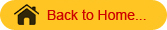Contingency table – 2x2 contingency table – Test for independence of attributes – test for goodness of fit of mendalian ratio

Test based on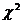-distribution

In case of attributes we can not employ the parametric tests such as F and t. Instead we have to applytest. When we want to test whether a set of observed values are in agreement with those expected on the basis of some theories or hypothesis. Thestatistic provides a measure of agreement between such observed and expected frequencies.

Chi-Square

Thetest has a number of applications. It is used to

• Test the independence of attributes
• Test the goodness of fit
• Test the homogeneity of variances
• Test the homogeneity of correlation coefficients
• Test the equaslity of several proportions.

In genetics it is applied to detect linkage.

Applications– test for goodness of fit

A very powerful test for testing the significance of the discrepancy between theory and experiment was given by Prof. Karl Pearson in 1900 and is known as “chi-square test of goodness of fit “.

If 0i, (i=1,2,…..,n) is a set of observed (experimental frequencies) and Ei (i=1,2,…..,n) is the corresponding set of expected (theoretical or hypothetical) frequencies, then,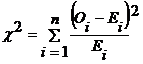It follows adistribution with n-1 d.f.  In case ofonly one tailed test is used.

Example

In plant genetics, our interest may be to test whether the observed segregation ratios deviate significantly from the mendelian ratios. In such situations we want to test the agreement between the observed and theoretical frequency, such test is called as test of goodness of fit.

Conditions for the validity of-test:-test is an approximate test for large values of ‘n’ for the validity of-test of goodness of fit between theory and experiment, the following conditions must be satisfied.

• The sample observations should be independent.

2. Constraints on the cell freqrequency, if any, should be linear.
Example: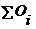=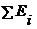.

3. N, the total frequency should be reasonably large, say greater then (>) 50.

4. No theoretical cell frequency should be less than (<)5. If any theoretical cell frequency is <5, then for the application of- test, it is pooled with the  preceding or scecceeding frequency so that the pooled frequency is more  than 5 and finally adjust for degree’s of freedom lost in pooling.

Example1
The number of yiest cells counted in a haemocytometer is compared to the theoretical value is given below. Does the experimental result support the theory?

 No. of Yeast cells in the square Obseved Frequency Expected Frequency 0 103 106 1 143 141 2 98 93 3 42 41 4 8 14 5 6 5

Solution
H0: the experimental results support the theory
H1: the esperimental results does not support the theory.
Level of significance=5%
Test Statistic: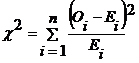Oi Ei Oi­-Ei (Oi­-Ei)2 (Oi­-Ei)2/Ei 103 106 -3 9 0.0849 143 141 2 4 0.0284 98 93 5 25 0.2688 42 41 1 1 0.0244 8 14 -6 36 2.5714 6 5 1 1 0.2000 400 400 3.1779

\=3.1779

Table value(6-1=5 at 5 % l.os)= 11.070
Inference<tab
We accept the null hypothesis.
(i.e) there is a good correspondence between theory and experiment.test for independence of attributes

At times we may consider two charactertistics on attributes simultaneously. Our interest will be to test the association between these two attributes
Example:- An entomologist may be interested to know the effectiveness of different concentrations of the chemical in killing the insects. The concentrations of chemical form one attribute. The state of insects ‘killed & not killed’ forms another attribute. The result of this experiment can be arranged in the form of a contingency table.  In general one attribute may be divided into m classes as A 1,A 2, …….A m  and the other attribute may be divided into  n classes as B 1,B 2, ……B n . Then the contingency table will have m x n cells. It is termed as m x n contingency table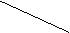A B A1 A2 … Aj … Am Row Total B1 O11 O12 … O1j O1m r1 B2 O21 O22 … O2j O2m r2 . . . Bi Oij Oi2 … Oij Oim ri . . . Bn On1 On2 … Onj Onm rk Column Total c1 c2 … cj … cm n=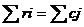where Oij’s are observed frequencies.
The expected frequencies corresponding to Oij is calculated as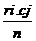. Theis computed as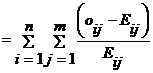where
Oij – observed frequencies
Eij – Expected frequencies
n= number of rows
m= number of columns
It can be verified that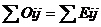Thisis distributed aswith (n-1) (m-1) d.f.

2x2 – contingency table

When the number of rows and numberof columns are equal to 2 it is termed as 2 x 2 contingency table .It will be in the following form

 B1                   B2 Row Total A1 A2 a                     b c                      d a+b       r1 c+d       r2 Column Total a+c                 b+d c1                     c2 a+b+c+d =n

Where a, b, c and d are cell frequancies c1 and c2 are column totals, r1 and r2 are row totals and n is the total number of observations.
In case of 2 x 2 contigency tablecan be directly found using the short cut formula,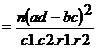The d.f  associated withis (2-1) (2-1) =1

Yates correction for continuity
If anyone of the cell frequency is < 5, we use Yates correction to makeas continuous. The yares correction is made by adding 0.5 to the least cell frequency and adjusting the other cell frequencies so that the column and row totals remain same . suppose, the firat cell frequency is to be corrected then the consigency table will be as follows:

 B1 B2 Row Total A1 A2 a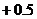b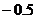a+b=r1 cdc+d =r2 Column Total a+c=c1 b+d=c2 n = a+b+c+d

Then  use the- statistic as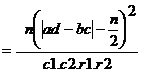The d.f  associated withis (2-1) (2-1) =1

Exapmle 2
The severity of a disease and blood group were studied in a research projest. The findings sre given in the following table, knowmn as the m xn contingency table. Can this severity of the condition and blood group are associated.
Severity of a disease classified by blood group in 1500 patients.

 Condition Blood Groups Total O A B AB Severe 51 40 10 9 110 Moderate 105 103 25 17 250 Mild 384 527 125 104 1140 Total 540 670 160 130 1500

Solution
H0: The severity of the disease is not associated with blood group.
H1: The severity of the disease is associated with blood group.
Calculation of Expected frequencies

 Condition Blood Groups Total O A B AB Severe 39.6 49.1 11.7 9.5 110 Moderate 90.0 111.7 26.7 21.7 250 Mild 410.4 509.2 121.6 98.8 1140 Total 540 670 160 130 1500

Test statistic:The d.f. associated with theis (3-1)(4-1) = 6
Calculations

 Oi Ei Oi­-Ei (Oi­-Ei)2 (Oi­-Ei)2/Ei 51 39.6 11.4 129.96 3.2818 40 49.1 -9.1 82.81 1.6866 10 11.7 -1.7 2.89 0.2470 9 9.5 -0.5 0.25 0.0263 105 90.0 15 225.00 2.5000 103 111.7 -8.7 75.69 0.6776 25 26.7 -1.7 2.89 0.1082 17 21.7 -4.7 22.09 1.0180 384 410.4 -26.4 696.96 1.6982 527 509.2 17.8 316.84 0.6222 125 121.6 3.4 11.56 0.0951 104 98.8 5.2 27.04 0.2737 Total 12.2347

\=12.2347
Table value offor 6 d.f. at 5% level of significance is 12.59
Inference<tab
We accept the null hypothesis.
The severity of the disease has no association with blood group.

Example 3
In order to determine the possible effect of a chemical treatment on the rate of germination of cotton seeds a pot culture experiment was conducted. The results are given below
Chemical treatment and germination of cotton seeds

 Germinated Not germinated Total Chemically Treated 118 22 140 Untreated 120 40 160 Total 238 62 300

Does the chemical treatrment improve the germination rate of cotton seeds?

Solution
H0:The chemical treatment does not improve the germination rate of cotton seeds.
H1: The chemical treatment improves the germination rate of cotton seeds.
Level of significance = 1%
Test statistic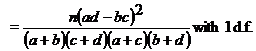Table value(1) d.f. at 1 % L.O.S = 6.635
Inference<tab
We accept the null hypothesis.
The chemical treatmentwill not  improve the germination rate of cotton seeds significantly.

Example 4
In an experiment on the effect of a growth regulator on fruit setting in muskmelon the following results were obtained. Test whether the fruit setting in muskmelon and the application of growth regulator are independent at 1% level.

 Fruit set Fruit not set Total Treated 16 9 25 Control 4 21 25 Total 20 30 50

Solution
H0:Fruit setting in muskmelon does not depend on the application of growth regulator.
H1: Fruit setting in muskmelon depend on the application of growth regulator.
Level of significance = 1%
After Yates correction we have

 Fruit set Fruit not set Total Treated 15.5 9.5 25 Control 4.5 20.5 25 Total 20 30 50

Tet statistic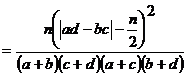Table value(1) d.f. at 1 % level of significance is 6.635
Inference>tab
We reject the null hypothesis.
Fruit setting in muskmelon is influenced by the  growth regulator. Application of growth regulator will increase fruit setting in musk melon.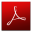Download this lecture as PDF here